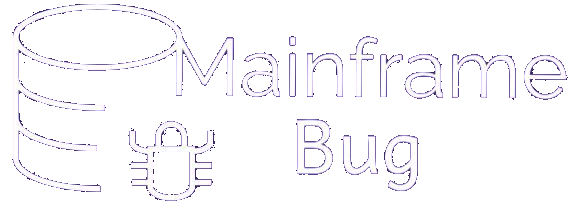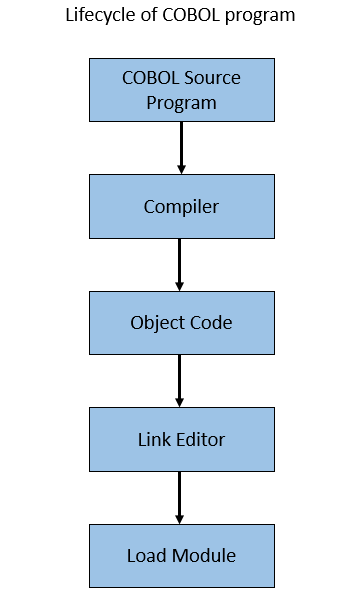## Module 9:- Compile, Link Edit and Run

### Lifecycle of COBOL program

COBOL program must be compiled and linked (in IBM terms, link edited) before it can be executed.Let’s understand each stages mentioned above

IMP NOTE: Example was given after these stages were explained, which will be helpful for practical purposes

#### COBOL Source program

COBOL program is typed at terminal using ISPF editor and usually stored as a member of PDS. This is called as source code.

#### Compiler & Object code

• Compiler act as language translator
• It’s role is to convert source code to relocatable object code
• It also supplies instructions necessary to debug a program if program is compiled with debug option
• Object code is in relocatable format with machine code that is not executable.
• Object code is usually stored in object library

General format of JCL that compiles program:-

Please look at Numberes in red, which is used for explanation later //JOBNAME JOB ACCTNO,NAME,MSGCLASS=1 (1) //STEPNAME EXEC PGM=IGYCRCTL,PARM=(OPTIONS) (2) //STEPLIB DD DSNAME= >IGY.V6R1M0 <.SIGYCOMP,DISP=SHR (3) // DD DSNAME=SYS1.SCEERUN,DISP=SHR // DD DSNAME=SYS1.SCEERUN2,DISP=SHR //SYSUT1 DD UNIT=SYSALLDA,SPACE=(SUBPARMS) (4) //SYSUT2 DD UNIT=SYSALLDA,SPACE=(SUBPARMS) //SYSUT3 DD UNIT=SYSALLDA,SPACE=(SUBPARMS) //SYSUT4 DD UNIT=SYSALLDA,SPACE=(SUBPARMS) //SYSUT5 DD UNIT=SYSALLDA,SPACE=(SUBPARMS) //SYSUT6 DD UNIT=SYSALLDA,SPACE=(SUBPARMS) //SYSUT7 DD UNIT=SYSALLDA,SPACE=(SUBPARMS) //SYSPRINT DD SYSOUT=A (5) //SYSLIN DD DSNAME=MYPROG,UNIT=SYSALLDA, (6) // DISP=(MOD,PASS),SPACE=(SUBPARMS) //SYSIN DD DSNAME=DSNAME,UNIT=DEVICE, (7) // VOLUME=(SUBPARMS),DISP=SHR

Explanation:-

We have marked numbers in above code snippet which will be referenced below for explanation purpose

(1) It is job statement to indicate start of job

(2) The EXEC statement indicating Enterprise COBOL compiler ‘IGYCRCTL’ is used

(3) DD statement states the dataset where the ‘IGYCRCTL’ mentioned in EXEC statement resides

(4) The SYSUT DD statements define the utility data sets that the compiler will use to process the source program. All SYSUT files must be on direct-access storage devices.

(5) Defined dataset that receives output from compiler options such as LIST and MAP

(6) The declaration SYSLIN DD defines the data set (the object module) receiving output from the OBJECT compiler option.

(7) [IMPORTANT] SYSIN DD is used to specify the dataset (COBOL source code) to be used as input to the job step

[Example for same is provided in next Article]

• Linkage editor or Link Editor combines the relocatable object code of source file with the relocatable object code of any pre-compiled subroutine or any external procedures that program may reference
• It converts Object module into Load module
• Load module is also relocatable, but with executable machine code
• The load module is what is called as executable code
• Through JCL one can execute COBOL program which is available in load module. i.e. you cannot execute COBOL program until it is converted into load module

General format of JCL that compiles program:-

Please look at Numberes in red, which is used for explanation later //LKED EXEC PGM=IEWL,REGION=1024K,PARM=(options) (1) //SYSPRINT DD SYSOUT=A (2) //SYSLIB DD DSN=COMMON.PDS.LIB1,DISP=SHR (3) // DD DSN=COMMON.PDS.LIB2,DISP=SHR //SYSLMOD DD DSN=ENDV.TEST.LOADLIB(MFPROG1), (4) // DISP=SHR //SYSUT1 DD UNIT=SYSALLDA,DCB=BLKSIZE=1024, (5) // SPACE=(CYL,(1,1)) //SYSTERM DD SYSOUT=* //SYSPRINT DD SYSOUT=* //SYSLIN DD DSN=&&LOADSET,DISP=(OLD,DELETE) (6) //SYSIN DD DUMMY

Explanation:-

We have marked numbers in above code snippet which will be referenced below for explanation purpose

(1) This step indicates that program = IEWL will be invoked

(2) The SYSPRINT DD statement defines the data set which receives the output.

(3) The SYSLIB statement identifies the name of the library or libraries that contains the object code.

(4) [IMPORTANT] SYSLMOD DD statement identifies dataset location, where load module to be stored

(5) IEWL will use these units to process object module

(6) [IMPORTANT]SYSLIN DD statement defines the dataset, we will give OBJECT MODULE here as an input to IEWL

[Example for same is provided in next Article]